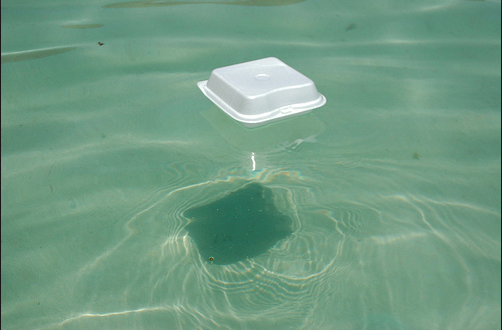# Floating boxA box with density $\rho = 800 \text{ kg/m}^3$ is floating face down on a fluid with density $\rho _{f} = 1200 \text{ kg/m}^{3}.$ If the box has a height of $H= 6.0 \text{ cm},$ by what depth $h$ is the box submerged?

The floating box is a rectangular parallelepiped.

×

Problem Loading...

Note Loading...

Set Loading...